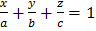# A variable plane is at a distance,from the origin and meets the coordinate axes inThen, the locus of the centroid ofis a)b)c)d)## Question ID - 54423 :- A variable plane is at a distance,from the origin and meets the coordinate axes inThen, the locus of the centroid ofis a)b)c)d)3537

(d)

Let the equation of the variable plane beThis meets the coordinate axes atandLetbe the coordinates of the centroid ofThen,…(i)

The plane is at a distance,from the originHence, the locus ofisNext Question :
 The ratio of a man’s age and his son’s age is 7:3 and the product of their ages is 756. The ratio of their ages after 6 years will be (a) 5:2 (b) 2:1 (c) 11:7 (d) 13:9